# Excel logical operators: equal to, not equal to, greater than, less than

Many tasks you perform in Excel involve comparing data in different cells. For this, Microsoft Excel provides six logical operators, which are also called comparison operators. This tutorial aims to help you understand the insight of Excel logical operators and write the most efficient formulas for your data analysis.

## Excel logical operators - overview

A logical operator is used in Excel to compare two values. Logical operators are sometimes called Boolean operators because the result of the comparison in any given case can only be either TRUE or FALSE.

Six logical operators are available in Excel. The following table explains what each of them does and illustrates the theory with formula examples.

 Condition Operator Formula Example Description Equal to = =A1=B1 The formula returns TRUE if a value in cell A1 is equal to the values in cell B1; FALSE otherwise. Not equal to <> =A1<>B1 The formula returns TRUE if a value in cell A1 is not equal to the value in cell B1; FALSE otherwise. Greater than > =A1>B1 The formula returns TRUE if a value in cell A1 is greater than a value in cell B1; otherwise it returns FALSE. Less than < =A1= =A1>=B1 The formula returns TRUE if a value in cell A1 is greater than or equal to the values in cell B1; FALSE otherwise. Less than or equal to <= =A1<=B1 The formula returns TRUE if a value in cell A1 is less than or equal to the values in cell B1; FALSE otherwise.

The screenshot below demonstrates the results returned by Equal to, Not equal to, Greater than and Less than logical operators: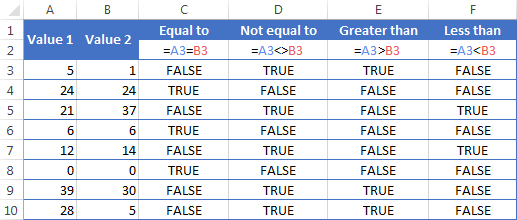It may seem that the above table covers it all and there's nothing more to talk about. But in fact, each logical operator has its own specificities and knowing them can help you harness the real power of Excel formulas.

### Using "Equal to" logical operator in Excel

The Equal to logical operator (=) can be used to compare all data types - numbers, dates, text values, Booleans, as well as the results returned by other Excel formulas. For example:

 =A1=B1 Returns TRUE if the values in cells A1 and B1 are the same, FALSE otherwise. =A1="oranges" Returns TRUE if cells A1 contain the word "oranges", FALSE otherwise. =A1=TRUE Returns TRUE if cells A1 contain the Boolean value TRUE, otherwise it returns FALSE. =A1=(B1/2) Returns TRUE if a number in cell A1 is equal to the quotient of the division of B1 by 2, FALSE otherwise.

#### Example 1. Using the "Equal to" operator with dates

You might be surprised to know that the Equal to logical operator cannot compare dates as easily as numbers. For example, if the cells A1 and A2 contain the date "12/1/2014", the formula `=A1=A2` will return TRUE exactly as it should.

However, if you try either `=A1=12/1/2014` or `=A1="12/1/2014"` you will get FALSE as the result. A bit unexpected, eh?

The point is that Excel stores dates as numbers beginning with 1-Jan-1900, which is stored as 1. The date 12/1/2014 is stored as 41974. In the above formulas, Microsoft Excel interprets "12/1/2014" as a usual text string, and since "12/1/2014" is not equal to 41974, it returns FALSE.

To get the correct result, you must always wrap a date in the DATEVALUE function, like this `=A1=DATEVALUE("12/1/2014")`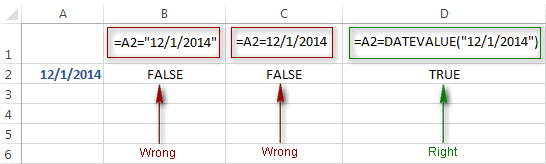Note. The DATEVALUE function needs to be used with other logical operator as well, as demonstrated in the examples that follow.

The same approach should be applied when you use Excel's equal to operator in the logical test of the IF function. You can find more info as well as a few formula examples in this tutorial: Using Excel IF function with dates.

#### Example 2. Using the "Equal to" operator with text values

Using Excel's Equal to operator with text values does not require any extra twists. The only thing you should keep in mind is that the Equal to logical operator in Excel is case-insensitive, meaning that case differences are ignored when comparing text values.

For example, if cell A1 contains the word "oranges" and cell B1 contains "Oranges", the formula `=A1=B1` will return TRUE.

If you want to compare text values taking in to account their case differences, you should use the EXACT function instead of the Equal to operator. The syntax of the EXACT function is as simple as:

EXACT(text1, text2)

Where text 1 and text2 are the values you want to compare. If the values are exactly the same, including case, Excel returns TRUE; otherwise, it returns FALSE. You can also use the EXACT function in IF formulas when you need a case-sensitive comparison of text values, as shown in the below screenshot: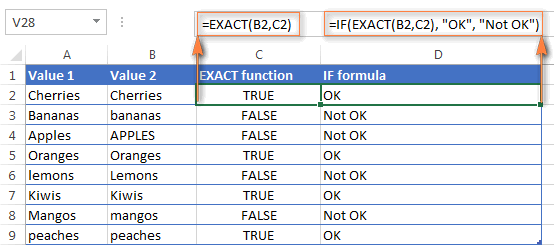Note. If you want to compare the length of two text values, you can use the LEN function instead, for example `=LEN(A2)=LEN(B2)` or `=LEN(A2)>=LEN(B2)`.

#### Example 3. Comparing Boolean values and numbers

There is a widespread opinion that in Microsoft Excel the Boolean value of TRUE always equates to 1 and FALSE to 0. However, this is only partially true, and the key word here is "always" or more precisely "not always" : )

When writing an 'equal to' logical expression that compares a Boolean value and a number, you need to specifically point out for Excel that a non-numeric Boolean value should be treated as a number. You can do this by adding the double minus sign in front of a Boolean value or a cell reference, e. g. `=A2=--TRUE` or `=A2=--B2`.

The 1st minus sign, which is technically called the unary operator, coerces TRUE/FALSE to -1/0, respectively, and the second unary negates the values turning them into +1 and 0. This will probably be easier to understand looking at the following screenshot: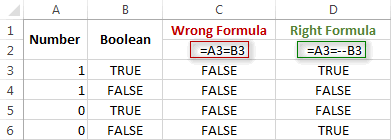Note. You should add the double unary operator before a Boolean when using other logical operators such as not equal to, greater than or less than to correctly compare a numeric and Boolean values.

When using logical operators in complex formulas, you might also need to add the double unary before each logical expression that returns TRUE or FALSE as the result. Here's an example of such a formula: SUMPRODUCT and SUMIFS in Excel.

### Using "Not equal to" logical operator in Excel

You use Excel's Not equal to operator (<>) when you want to make sure that a cell's value is not equal to a specified value. The use of the Not equal to operator is very similar to the use of Equal to that we discussed a moment ago.

The results returned by the Not equal to operator are analogous to the results produced by the Excel NOT function that reverses the value of its argument. The following table provides a few formula examples.

 Not equal to operator NOT function Description =A1<>B1 =NOT(A1=B1) Returns TRUE if the values in cells A1 and B1 are not the same, FALSE otherwise. =A1<>"oranges" =NOT(A1="oranges") Returns TRUE if cell A1 contains any value other than "oranges", FALSE if it contains "oranges" or "ORANGES" or "Oranges", etc. =A1<>TRUE =NOT(A1=TRUE) Returns TRUE if cell A1 contains any value other than TRUE, FALSE otherwise. =A1<>(B1/2) =NOT(A1=B1/2) Returns TRUE if a number in cell A1 is not equal to the quotient of the division of B1 by 2, FALSE otherwise. =A1<>DATEVALUE("12/1/2014") =NOT(A1=DATEVALUE("12/1/2014")) Returns TRUE if A1 contains any value other than the date of 1-Dec-2014, regardless of the date format, FALSE otherwise.

### Greater than, Less than, Greater than or equal to, Less than or equal to

You use these logical operators in Excel to check how one number compares to another. Microsoft Excel provides 4 comparison operates whose names are self-explanatory:

• Greater than (>)
• Greater than or equal to (>=)
• Less than (<)
• Less than or equal to (<=)

Most often, Excel comparison operators are used with numbers, date and time values. For example:

 =A1>20 Returns TRUE if a number in cell A1 is greater than 20, FALSE otherwise. =A1>=(B1/2) Returns TRUE if a number in cell A1 is greater than or equal to the quotient of the division of B1 by 2, FALSE otherwise. =A1

#### Using Excel comparison operators with text values

In theory, you can also use the greater than, greater than or equal to operators as well as their less than counterparts with text values. For example, if cell A1 contains "apples" and B1 contains "bananas", guess what the formula `=A1>B1` will return? Congratulations to those who've staked on FALSE : )

When comparing text values, Microsoft Excel ignores their case and compares the values symbol by symbol, "a" being considered the lowest text value and "z" - the highest text value.

So, when comparing the values of "apples" (A1) and "bananas" (B1), Excel starts with their first letters "a" and "b", respectively, and since "b" is greater than "a", the formula `=A1>B1` returns FALSE.

If the first letters are the same, then the 2nd letters are compared, if they happen to be identical too, then Excel gets to the 3rd, 4th letters and so on. For example, if A1 contained "apples" and B1 contained "agave", the formula `=A1>B1` would return TRUE because "p" is greater than "g".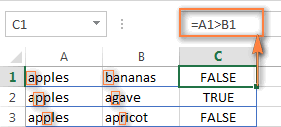At first sight, the use of comparison operators with text values seems to have very little practical sense, but you never know what you might need in the future, so probably this knowledge will prove helpful to someone.

## Common uses of logical operators in Excel

In real work, Excel logical operators are rarely used on their own. Agree, the Boolean values TRUE and FALSE they return, though very true (excuse the pun), are not very meaningful. To get more sensible results, you can use logical operators as part of Excel functions or conditional formatting rules, as demonstrated in the below examples.

#### 1. Using logical operators in arguments of Excel functions

When it comes to logical operators, Excel is very permissive and allows using them in parameters of many functions. One of the most common uses is found in Excel IF function where the comparison operators can help to construct a logical test, and the IF formula will return an appropriate result depending on whether the test evaluates to TRUE or FALSE. For example:

`=IF(A1>=B1, "OK", "Not OK")`

This simple IF formula returns OK if a value in cell A1 is greater than or equal to a value in cell B1, "Not OK" otherwise.

And here's another example:

`=IF(A1<>B1, SUM(A1:C1), "")`

The formula compares the values in cells A1 and B1, and if A1 is not equal to B1, the sum of values in cells A1:C1 is returned, an empty string otherwise.

Excel logical operators are also widely used in special IF functions such as SUMIF, COUNTIF, AVERAGEIF and their plural counterparts that return a result based on a certain condition or multiple conditions.

You can find a wealth of formula examples in the following tutorials:

#### 2. Using Excel logical operators in mathematical calculations

Of course, Excel functions are very powerful, but you don't always have to use them to achieve the desired result. For example, the results returned by the following two formulas are identical:

IF function: `=IF(B2>C2, B2*10, B2*5)`

Formula with logical operators: `=(B2>C2)*(B2*10)+(B2<=C2)*(B2*5)`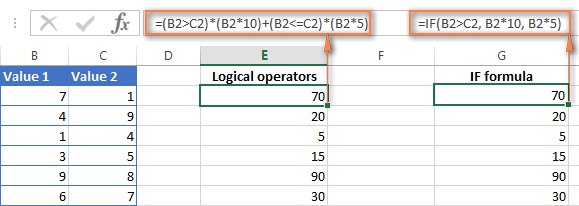I guess the IF formula is easier to interpret, right? It tells Excel to multiply a value in cell B2 by 10 if B2 is greater than C2, otherwise the value in B1 is multiplied by 5.

Now, let's analyze what the 2nd formula with the greater than and less than or equal to logical operators does. It helps to know that in mathematical calculations Excel does equate the Boolean value TRUE to 1, and FALSE to 0. Keeping this in mind, let's see what each of the logical expressions actually returns.

If a value in cell B2 is greater than a value in C2, then the expression B2>C2 is TRUE, and consequently equal to 1. On the other hand, B2<=C2 is FALSE and equal to 0. So, given that B2>C2, our formula undergoes the following transformation: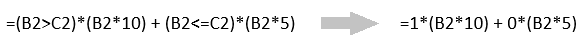Since any number multiplied by zero gives zero, we can cast away the second part of the formula after the plus sign. And because any number multiplied by 1 is that number, our complex formula turns into a simple =B2*10 that returns the product of multiplying B2 by 10, which is exactly what the above IF formula does : )

Obviously, if a value in cell B2 is less than in C2, then the expression B2>C2 evaluates to FALSE (0) and B2<=C2 to TRUE (1), meaning that the reverse of the described above will occur.

#### 3. Logical operators in Excel conditional formatting

Another common use of logical operators is found in Excel Conditional Formatting that lets you quickly highlight the most important information in a spreadsheet.

For example, the following simple rules highlight selected cells or entire rows in your worksheet depending on a value in column A:

Less than (orange): `=A1<5`

Greater than (green): `=A1>20`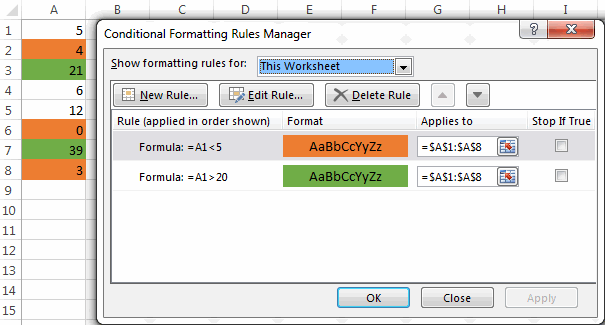For the detailed-step-by-step instructions and rule examples, please see the following articles:

As you see, the use of logical operators in Excel is intuitive and easy. In the next article, we are going to learn the nuts and bolts of Excel logical functions that allow performing more than one comparison in a formula. Please stay tuned and thank you for reading!

### 1,080 responses to "Excel logical operators: equal to, not equal to, greater than, less than"

1.mdc says:

Hi,

What formula should I use for:
If the value is not equal to "follow" in C4 then get the value in C4.

Thanks!

•Alexander Trifuntov (Ablebits.com Team) says:

Hello!

=IF(C4<>"follow",C4,"")

2.SIVA K says:

1+21=22 i need formula of round off one digit 22 = round on 20
2+34=36 this is round on = 40

3.AVI says:

I HAVE THREE SHEET, WITH SAME HEADER BUT VARIOUS VALUE IN IT, HOW TO KNOW WHICH SHEET DATA LESS THEN OUT OF THREE. CAN ANY OPTION OR COMMAND TO PRINT LESS SHEET NAME

SHEET NAME
1. 3338 DATA A 1620
2. 5498 DATA A 1550
3. 5913 DATA A 1820

4.April says:

I have a formula that I am stuck building It is using the below information. Can you please advise how to build the "if then" formula cell C5 for the below? I am stuck on trying to get the in between numbers to work. so far I have gotten = IF(C5<4,5,(C5

5 = 0 to 4
4 = 4.01 to 12
3 = 12.01 to 24
2 = 24.01 to 28
1 = 28<

5.GD says:

I have huge data with different Values and have to derive at some classification based on three data sets.

Col A & B has Numbers as Values and Col C has Names as Values

Col A has Values ranging from 0 to 1000
Col B has Values ranging from 0 to 1000000
Col C has Values WHITE, RED, ORANGE, BLUE

Could anyone help me to get this please. Below is an example of what I am looking for.

if A is greater than 1 and equals to 4 and is WHITE or RED term it as Medium
if B is greater than 8999 and equals to 16999 term it as Medium

6.Eric says:

Formula to return cell (eg A21 value) if value lies between >X<Y where X&Y are cell values. Please! Thanks

7.sofian says:

Hi there,

I have some excel question and below is some examples of the question.

I have a pallet and I would like to arrange my box on the this pallet. My Box stacking is not exceed more than 5 feet.

Pallet size is 1100x110x130 in mm.
Box size is 406x345x258 in mm

Base on size dimension above, manual calculation is as below

X : 1100 / 406 = 2.709 (this means only 2 box I only able to arrange in X direction)
Y : 1100 /345 = 3.188 (this means only 3 box I only able to arrange in Y direction)
Z :[ (5ft x 12 x 25.4) - 130] / 258 = 5.403 (this means only able to arrange the Box in 5 layer, Z direction)

Total Box in one pallet is = 2 x 3 x 5
= 30 boxes / pallet

The question is how can I change the answer 2.709 become 2 using excel equation?

8.John Lim says:

Hi everyone, need help on this problem.

A1 = Karen (Name of Tenant)
B1 = Total Monthly Rental Payment
C1 = Rental Rate per month - 10,000.00
E1 = Less EWT 5%
F1 = PEZA accredited

i want that result on B1 should be non-VAT 12% exclusive if the tenant is PEZA accredited

pls. help me on my current formula

current formula: =(I5+G5)-H5

9.PJ says: